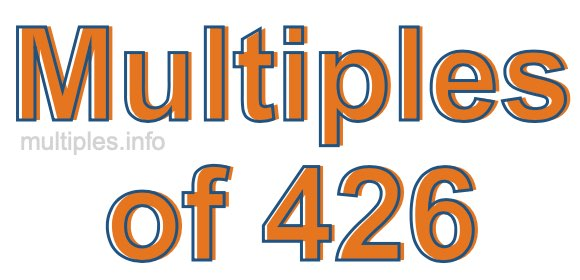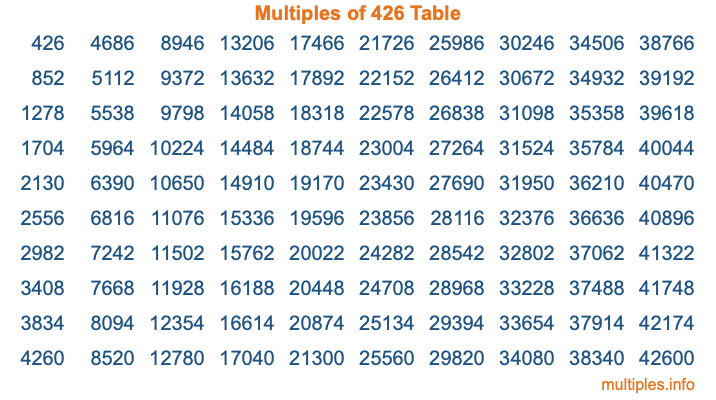Multiples of 426Welcome to the Multiples of 426 page. Here we will first teach you everything you will ever need to know about the multiples of 426, and then give you a study guide summary of everything we taught you to make sure you remember it all. Use this page to look up facts and learn information about the multiples of 426. This page will make you a multiples of four hundred twenty-six expert!

Definition of Multiples of 426
Multiples of 426 are all the numbers that when divided by 426 equal an integer. Each of the multiples of 426 are called a multiple. A multiple of 426 is created by multiplying 426 by an integer.

Therefore, to create a list of multiples of 426, you start with 1 multiplied by 426, then 2 multiplied by 426, then 3 multiplied by 426, and so on for as long as you want. Thus, the list of the first five multiples of 426 is 426, 852, 1278, 1704, and 2130. To see a larger list of multiples of 426, see the printable image of Multiples of 426 further down on this page. We also have a category where you can choose any nth multiple of 426.

Multiples of 426 Checker
The Multiples of 426 Checker below checks to see if any number of your choice is a multiple of 426. In other words, it checks to see if there is any number (integer) that when multiplied by 426 will equal your number. To do that, we divide your number by 426. If the the quotient is an integer, then your number is a multiple of 426.

Is  a multiple of 426?

Least Common Multiple of 426 and ...
A Least Common Multiple (LCM) is the lowest multiple that two or more numbers have in common. This is also called the smallest common multiple or lowest common multiple and is useful to know when you are adding our subtracting fractions. Enter one or more numbers below (426 is already entered) to find the LCM.

Check out our LCM Calculator if you need more details about the Least Common Multiple or if you need the LCM for different numbers for adding and subtraction fractions.

nth Multiple of 426
As we stated above, 426 is the first multiple of 426, 852 is the second multiple of 426, 1278 is the third multiple of 426, and so on. Enter a number below to find the nth multiple of 426.

th multiple of 426

Multiples of 426 vs Factors of 426
426 is a multiple of 426 and a factor of 426, but that is where the similarities end. All postive multiples of 426 are 426 or greater than 426. All positive factors of 426 are 426 or less than 426.

Below is the beginning list of multiples of 426 and the factors of 426 so you can compare:

Multiples of 426: 426, 852, 1278, 1704, 2130, etc.

Factors of 426: 1, 2, 3, 6, 71, 142, 213, 426

As you can see, the multiples of 426 are all the numbers that you can divide by 426 to get a whole number. The factors of 426, on the other hand, are all the whole numbers that you can multiply by another whole number to get 426.

It's also interesting to note that if a number (x) is a factor of 426, then 426 will also be a multiple of that number (x).

Multiples of 426 vs Divisors of 426
The divisors of 426 are all the integers that 426 can be divided by evenly. Below is a list of the divisors of 426.

Divisors of 426: 1, 2, 3, 6, 71, 142, 213, 426

The interesting thing to note here is that if you take any multiple of 426 and divide it by a divisor of 426, you will see that the quotient is an integer.

Multiples of 426 Table
Below is an image of the first 100 multiples of 426 in a table. The table is in chronological order, column by column. The first column has the first ten multiples of 426, the second column has the next ten multiples of 426, and so on.The Multiples of 426 Table is also referred to as the 426 Times Table or Times Table of 426. You are welcome to print out our table for your studies.

Negative Multiples of 426
Although not often discussed or needed in math, it is worth mentioning that you can make a list of negative multiples of 426 by multiplying 426 by -1, then by -2, then by -3, and so on, to get the following list of negative multiples of 426:

-426, -852, -1278, -1704, -2130, etc.

Multiples of 426 Summary
Below is a summary of important Multiples of 426 facts that we have discussed on this page. To retain the knowledge on this page, we recommend that you read through the summary and explain to yourself or a study partner why they hold true.

There are an infinite number of multiples of 426.

A multiple of 426 divided by 426 will equal a whole number.

426 divided by a factor of 426 equals a divisor of 426.

The nth multiple of 426 is n times 426.

The largest factor of 426 is equal to the first positive multiple of 426.

426 is a multiple of every factor of 426.

426 is a multiple of 426.

A multiple of 426 divided by a divisor of 426 equals an integer.

426 divided by a divisor of 426 equals a factor of 426.

Any integer times 426 will equal a multiple of 426.

Multiples of a Number
Here you can get the multiples of another number, all with the same attention to detail as we did for multiples of 426 on this page.

Multiples of
Multiples of 427
Did you find our page about multiples of four hundred twenty-six educational? Do you want more knowledge? Check out the multiples of the next number on our list!